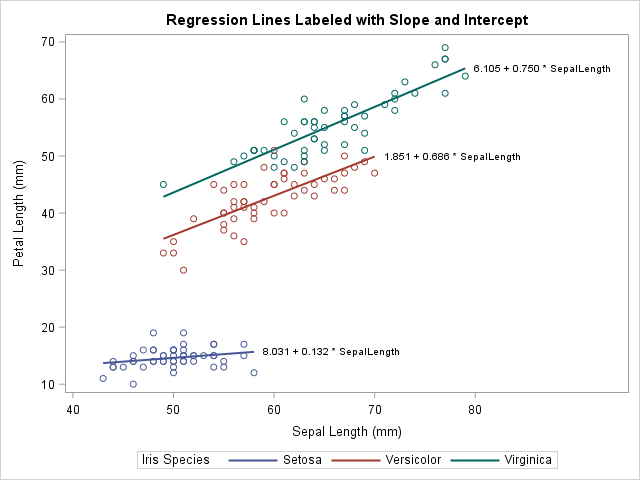A SAS programmer asked how to label multiple regression lines that are overlaid on a single scatter plot. Specifically, he asked to label the curves that are produced by using the REG statement with the GROUP= option in PROC SGPLOT. He wanted the labels to be the slope and intercept of a linear regression line, as shown to the right. (Click to enlarge.)

Initially I thought that you could use the CURVELABEL option on the REG statement to generate labels, as follows:

```proc sgplot data=sashelp.iris noautolegend; reg x=SepalLength y=PetalLength / group=Species CURVELABEL; /* does NOT work */ run;```

However, the SAS log displays the following warning:

```WARNING: CURVELABEL not supported for fit plots when a group variable is used. The option will be ignored.```

Fortunately, I thought of two other ways to create a graph that has a regression line for each group level, each with its own label. For linear regression, you can use the LINEPARM statement, as shown in the article "Add a diagonal line to a scatter plot." For general (possibly nonlinear) regression curves, you can find the location of the end of the curve and use the TEXT statement in PROC SGPLOT to add a label at that location.

### Label the regression line for each group: The LINEPARM statement

Let's use Fisher's Iris data set for our example data. The Iris data contains 50 observations for each of three species of flowers: iris Setosa, iris Versicolor, and iris Virginica. The programmer wants to label the regression line for each species by using the slope and intercept of the line. The first step is to create a SAS data set that contains the intercept and slope for each curve. You can use the OUTEST= option in PROC REG to write the parameter estimates (intercept and slope) to a SAS data set. You can then use the CATX function in the DATA step to construct the labels, as follows:

```proc sort data=sashelp.iris out=iris; by Species; run;   /* compute parameter estimates */ proc reg data=iris outest=PE noprint; by Species; model PetalLength = SepalLength; run;   /* construct labels from the parameter estimates */ data Labels; length Label \$30; set PE(rename=(SepalLength=Slope)); /* independent variable */ Label = catx(" ", put(Intercept, BestD5.), '+', /* separate by blank */ put(Slope, BestD5.), '* SepalLength'); keep Label Species Intercept Slope; run;   proc print noobs; run;```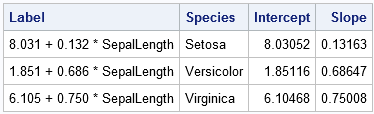The LABELS data set contains a label for the regression line in each group. You can use other labels if you prefer. The following DATA step combines the labels with the original data. The SCATTER statement in PROC SGPLOT displays the data. The LINEPARM statement draws the lines and adds labels to the end of each line.

```data Plot; set iris Labels; run;   title "Regression Lines Labeled with Slope and Intercept"; proc sgplot data=Plot; scatter x=SepalLength y=PetalLength / group=Species; lineparm x=0 y=Intercept slope=Slope / group=Label curvelabel curvelabelloc=outside clip; run;```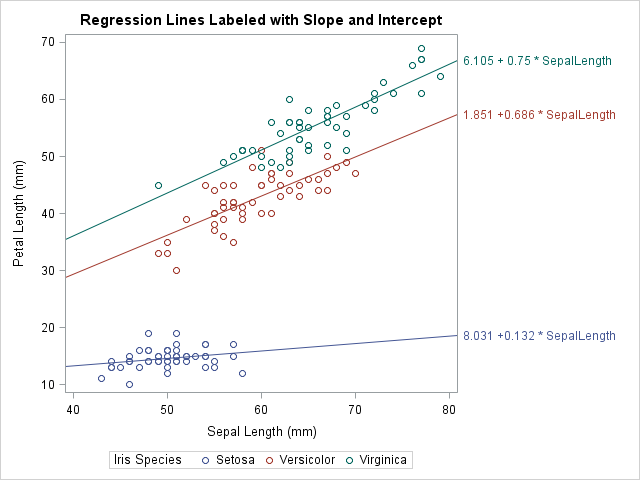Success! The regression line for each group is labeled by the formula for the line. For more information about displaying the formula for a regression line, see the SAS/STAT example "Adding Equations and Special Characters to Fit Plots."

### Label the regression line for each group: The TEXT statement

The preceding method uses the LINEPARM statement, so it only works for lines. However, the user actually wanted to use the REG statement. With a little work, you can label curves that are produced by the REG statement or other curve-fitting statements. The idea is to obtain the data coordinates for the end of the curve, which will become the location of the label.

You might be thinking, if the curve is produced by a regression statement in PROC SGPLOT, how can we get the data coordinates out of the plot and into a data set? The answer is simple: You can use the ODS OUTPUT statement to write a data set that contains the data in any ODS graph. You can apply this trick to any ODS graph, including graphs created by SGPLOT. The following call to PROC SGPLOT uses an ODS OUTPUT statement to create a SAS data set that contains the data in the regression plot:

```/* use ODS OUTPUT to find data coordinates of end of lines */ proc sgplot data=iris; ods output sgplot=RegPlot; /* name of ODS table is 'sgplot' */ reg x=SepalLength y=PetalLength / group=Species; run;   proc contents short varnum; run; /* find names used by graph */```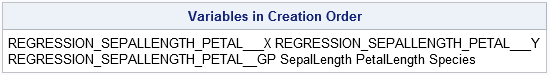The variable names that are automatically manufactured by SAS procedures can be long and unwieldy, as shown by the call to PROC CONTENTS. I usually rename the long names to simpler names such as X, Y, GROUP, and so on. You should look at the structure of the REGPLOT data set so that the next DATA step makes sense. The DATA step saves only the last coordinates along the curve for each group (species).

```data Coords; set RegPlot( rename=(REGRESSION_SEPALLENGTH_PETAL___X = x REGRESSION_SEPALLENGTH_PETAL___Y = y REGRESSION_SEPALLENGTH_PETAL__GP = Group) where=(x ^=.)); by Group; if last.Group; keep x y Group; run;   proc print noobs; run;```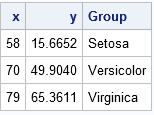The COORDS data contains the location (in data coordinates) of the end of each regression line. You can overlay labels at these coordinates to label the curves. From the preceding section, the labels are in the LABELS data set, so you can merge the two data sets, as follows:

```/* combine the positions and labels with original data */ data A; merge Labels Coords(rename=(Group=Species)); by Species; run;   data Plot; set iris A; /* optional: pad label with blanks on the left (if length is long enough) */ Label = " " || Label; run;   proc sgplot data=Plot; reg x=SepalLength y=PetalLength / group=Species; text x=x y=y text=Label / position=right; run;```

The graph is shown at the top of this article.

### Label multiple regression curves

If you study the previous section, you will see that the code does not rely on the linearity of the regression model. The same code works for polynomial regression and nonparametric regression curves such as are created by the LOESS and PBSPLINE statements in PROC SGPLOT. The following graph shows a PBSPLINE fit to the IRIS data. Because the penalized B-spline curve is nonparametric, there is no equation to display as a label. Instead, I use the Species name as a label and suppress the legend at the bottom of the graph. You can download the SAS program that creates this and all the graphs in this article.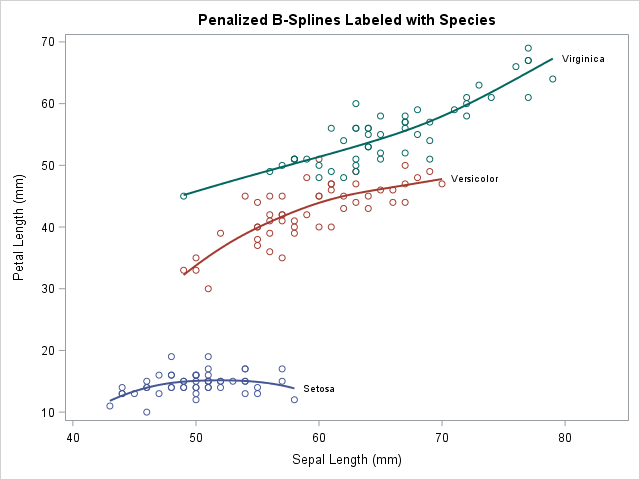The post Label multiple regression lines in SAS appeared first on The DO Loop.